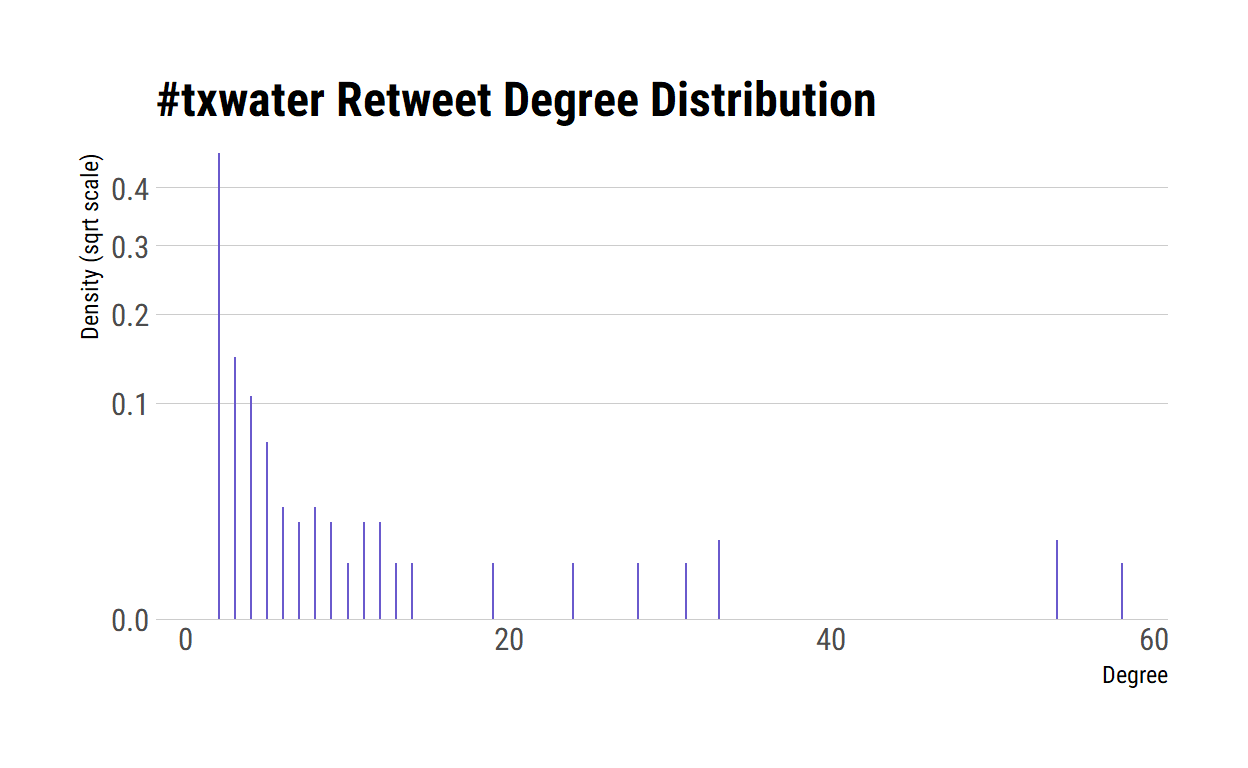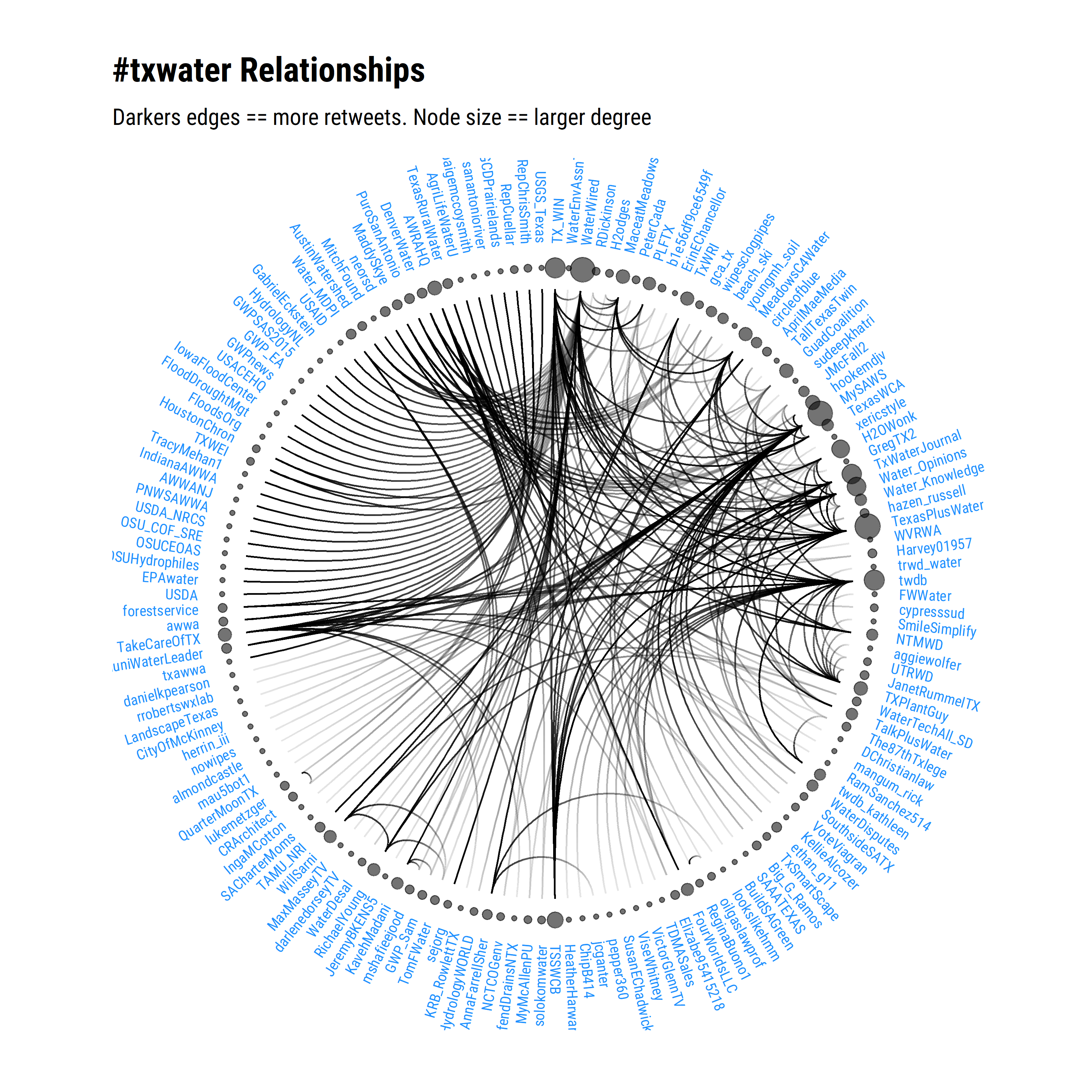# txwater retweets

Let’s find out the retweet relationships for txwater twitter users.

Michael Schramm https://michaelpaulschramm.com (Texas Water Resources Institute)https://twri.tamu.edu
February 21,2018

Note, post updated on July 29, 2020.

Let’s find out the retweet relationships for #txwater twitter users. I’m going to use the R recipes by Bob Rudis in 21 Recipes for Mining Twitter Data with rtweet.

## Extract the origin

``````
library(rtweet)
library(tidyverse)
txwater <- search_tweets("#txwater",
n = 1000,
token = token)
output <- filter(txwater,
retweet_count > 0) %>%
select(text,
mentions_screen_name,
retweet_count) %>%
mutate(text = substr(text, 1, 30)) %>%
unnest()
as_tibble(output)``````
``````
# A tibble: 378 x 3
text                     mentions_screen_n~ retweet_count
<chr>                    <chr>                      <int>
1 "We appreciate the oppo~ TexasPlusWater                 2
2 "We appreciate the oppo~ txawwa                         2
3 "We appreciate the oppo~ TexasWCA                       2
4 "Houston Officials Are ~ <NA>                           2
5 "This issue of @MuniWat~ TexasWCA                       5
6 "This issue of @MuniWat~ MuniWaterLeader                5
7 "This issue of @MuniWat~ NTMWD                          5
8 "This issue of @MuniWat~ MySAWS                         5
9 "The Texas Parks and Wi~ <NA>                           2
10 "Frankly the change in ~ PLFTX                          1
# ... with 368 more rows``````

## Plot txwater retweet degree distribution

``````
library(igraph)
library(hrbrthemes)
rt_g <- filter(txwater, retweet_count > 0) %>%
select(screen_name, mentions_screen_name) %>%
unnest(mentions_screen_name) %>%
filter(!is.na(mentions_screen_name)) %>%
graph_from_data_frame()
ggplot(data_frame(y=degree_distribution(rt_g), x=1:length(y))) +
geom_segment(aes(x, y, xend=x, yend=0), color="slateblue") +
scale_y_continuous(expand=c(0,0), trans="sqrt") +
labs(x="Degree", y="Density (sqrt scale)", title="#txwater Retweet Degree Distribution") +
theme_ipsum_rc(grid="Y", axis="x")``````## Plot retweet relationships

``````
library(ggraph)
# Label nodes
V(rt_g)\$node_label <- unname(names(V(rt_g)))
# Size of node
V(rt_g)\$node_size <- unname(ifelse(degree(rt_g)[V(rt_g)] > 1, degree(rt_g), 1))
# Adjust angle of label based on position
nIds <- length(V(rt_g))
V(rt_g)\$Id <- seq(1:nIds)
V(rt_g)\$label_angle <- 90 - 360 *  V(rt_g)\$Id / nIds
V(rt_g)\$hjust <- ifelse(V(rt_g)\$label_angle < -90, 1, 0)
# Flip text depending on what side of the plot it is on
V(rt_g)\$angle <- ifelse(V(rt_g)\$label_angle < -90, V(rt_g)\$label_angle+180, V(rt_g)\$label_angle)
p <- ggraph(rt_g, layout = 'linear', circular = TRUE) +
geom_edge_arc(aes(alpha=..index..)) +
geom_node_point(aes(x = x*1.07, y=y*1.07, size=node_size,  alpha=0.2)) +
geom_node_text(aes(x=x*1.15, y=y*1.15,label=node_label, angle=angle, hjust=hjust),
color="dodgerblue", size=2.7, family=font_rc) +
coord_fixed() +
labs(title="#txwater Relationships", subtitle="Darkers edges == more retweets. Node size == larger degree") +
theme_graph(base_family=font_rc) +
theme(legend.position="none") +
expand_limits(x = c(-1.3, 1.3), y = c(-1.3, 1.3))
p``````The `rtweet` `search_tweets()` function returns approximately 8 days worth of retweets. Depending on when this script was run, the relationships might change. I was suprised to see only a handful of state agencies are well engaged in this hashtag. The next step is to take look at relationships between users and text sentiment analysis. But that is it for this post.

### Corrections

If you see mistakes or want to suggest changes, please create an issue on the source repository.

### Reuse

Text and figures are licensed under Creative Commons Attribution CC BY 4.0. Source code is available at https://github.com/mps9506/mschramm, unless otherwise noted. The figures that have been reused from other sources don't fall under this license and can be recognized by a note in their caption: "Figure from ...".

### Citation

For attribution, please cite this work as

`Schramm (2018, Feb. 21). @mpschramm: txwater retweets. Retrieved from https://michaelpaulschramm.com/posts/txwater_retweets/`

BibTeX citation

```@misc{schramm2018txwater,
author = {Schramm, Michael},
title = {@mpschramm: txwater retweets},
url = {https://michaelpaulschramm.com/posts/txwater_retweets/},
year = {2018}
}```